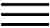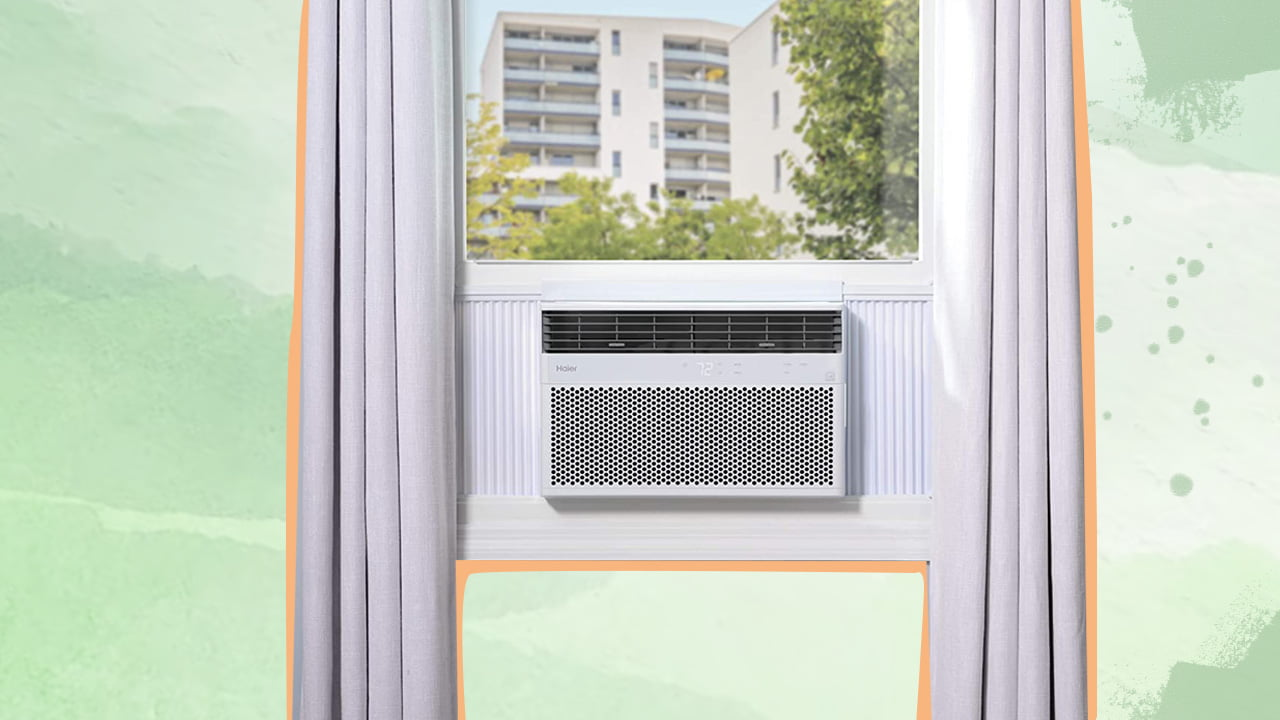X# Convert EER To COP And COP To EER (Calculator)To convert EER to COP, simply divide the value by 3.413. Accordingly, to convert COP to EER, multiply the value by 3.413. With this two-step calculation, you can easily measure how much cooling/heating a device produces as opposed to the electrical energy used in the process.

## Convert EER To COP or COP To EER Calculator

EER to COP Converter

Switch

COP to EER Converter

Switch

*Press the switch on the calculator to change the input from EER to COP and COP to EER with ease.

Energy efficiency is the fad now that people have finally understood that climate change is real!

From heating or cooling a room to powering different home appliances – you want to ensure you’re making the most out of your energy use. And that’s when the Energy-Efficiency Ratio (EER) and Coefficient Of Performance (COP) of your heating, ventilation, and air conditioning (HVAC) systems come into play.

These measures are used to determine the amount of energy consumed to produce a certain amount of heating or cooling output. However, while EER can be found in most specification sheets, COP cannot.

Fret not because converting EER to COP and vice versa is no rocket science. Here’s how…

## What Is Energy Efficiency Ratio (EER)?The EER of an air conditioner determines its cooling efficiency. It can be calculated by dividing the amount of cooling output (in British Thermal Units or BTUs) per hour by the amount of energy input (in watts) per hour. Simply put, EER measures the amount of cooling provided per unit of energy consumed. So, a higher EER indicates a more efficient air conditioning system.

Notably, EER is commonly used in the United States, whereas COP is a common unit in Europe and other parts of the world.

## What Is Coefficient Of Performance (COP)?

The COP measures the heating efficiency of heat pumps or the amount of heat produced per unit of energy consumed. The formula involves dividing the amount of heating output (in BTUs) per hour by the amount of energy input (in watts) per hour. As such, a higher COP implies a more efficient heat pump system.

Although the motive of both EER and COP is the same, i.e., measuring energy efficiency, the units are not directly comparable. This is because both consider different types of energy usage and include different units of measurement in the formula. Hence, you must use an online calculator to convert COP to EER and vice versa or check out the following formula.

## Convert EER To COP

EER / 3.413 = COP

The formula is derived from the fact that one wall equals 3.413 BTUs per hour. So, by dividing the EER by 3.413, we can convert it to the equivalent COP value.

For instance, if you own an air conditioner with an EER of 12, you can divide it by 3.413 to get its COP equivalent. Here, the approximate COP is 3.52.

## Convert COP To EER

COP x 3.143 = EER

As you may already notice, this formula is simply the reverse of the previous one. So, based on the same conversion factor, if you have a heat pump with a COP of 4.2, you can multiply the value by 3.143 to get its EER equivalent. Here, the resulting EER would be approximately 14.32.

COP ValueEER Rating (Calculated)
1.0 COP3.41 EER
1.5 COP5.12 EER
2.0 COP6.82 EER
2.5 COP8.53 EER
3.0 COP10.23 EER
3.5 COP11.94 EER
4.0 COP13.64 EER
4.5 COP15.35 EER
5.0 COP17.05 EER## Final Words

Converting EER to COP and COP to EER is a straightforward process that requires minimal calculation. However, it is still more convenient to use an online calculator, as all you have to do is input the values. As such, you must ensure that you add the correct values in the correct units.

Further, you should consider other factors, like the size of the system and its operating conditions, when selecting a device that’s both efficient and effective in meeting your requirements. You could also check the Seasonal Energy Efficiency Ratio (SEER) when buying a cooling system to determine how efficiently it works over an entire season.